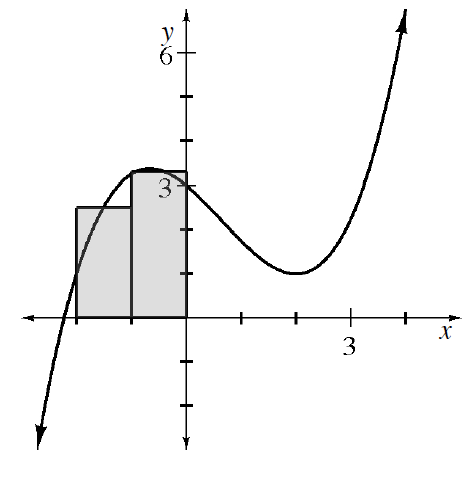### Home > APCALC > Chapter 1 > Lesson 1.4.1 > Problem1-145

1-145.

Cynthia began to draw midpoint rectangles to approximate the area under the curve for $−2 ≤ x ≤ 4$ given $f(x) = \frac { 1 } { 4 }x^3 −\frac { 1 } { 2 }x^2 − x + 3$. Trace Cynthia’s graph and finish drawing the remaining four midpoint rectangles. Then, compute the estimated area.

Make the remaining rectangles. Then calculate the areas of each rectangle and add them together to find $A(f, -2 ≤ x ≤ 4)$.

The width of all of the rectangles is $1$.